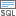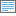# 条件测试 (Spatial Analyst)

## 使用情况

• 条件测试可通过 Where 子句中的 SQL 表达式进行指定。

• Where 子句使用 SQL 查询。 有关创建查询的详细信息，请参阅以下主题：

• 要在 Python 中使用 {where_clause}，应以引号括起来。 例如，"Value > 5000”

有关使用 Python 指定查询的详细信息，请参阅帮助。

• 如果输入是多波段栅格，则输出也将为多波段栅格。 该工具将在输入中的每个波段上执行操作。

• 在 ArcPy 中，如果输入是多维栅格，则将处理所有变量的所有切片，而输出将为多维栅格。

• 有关适用于此工具的地理处理环境的详细信息，请参阅分析环境和 Spatial Analyst

## 参数

 标注 说明 数据类型 输入栅格 基于逻辑表达式在其上执行布尔评估的输入栅格。 Raster Layer Where 子句 用于确定哪些输入像元将返回“真”值 (1) 以及哪些输入像元将返回“假”值 (0) 的逻辑表达式。Where 子句遵循 SQL 表达式的一般格式。 如果您单击编辑 SQL 模式按钮，则可以直接输入，例如 VALUE > 100。 如果处于编辑子句模式中，则可以通过单击添加子句模式按钮来开始构建表达式。 SQL Expression

### 返回值

 标注 说明 数据类型 输出栅格 输出栅格。输出像元值为 0 或 1。 Raster

`Test(in_raster, where_clause)`
 名称 说明 数据类型 in_raster 基于逻辑表达式在其上执行布尔评估的输入栅格。 Raster Layer where_clause 用于确定哪些输入像元将返回“真”值 (1) 以及哪些输入像元将返回“假”值 (0) 的逻辑表达式。表达式遵循 SQL 表达式的一般格式。 where_clause 的示例为 "VALUE > 100"。 SQL Expression

### 返回值

 名称 说明 数据类型 out_raster 输出栅格。输出像元值为 0 或 1。 Raster

### 代码示例

Test 示例 1（Python 窗口）

``````import arcpy
from arcpy import env
from arcpy.sa import *
env.workspace = "C:/sapyexamples/data"
outTest = Test("degs", "VALUE > 100")
outTest.save("C:/sapyexamples/output/outest.img")``````
Test 示例 2（独立脚本）

``````# Name: Test_Ex_02.py
# Description: Perform a Boolean evaluation of the input raster based
#              on a where clause
# Requirements: Spatial Analyst Extension

# Import system modules
import arcpy
from arcpy import env
from arcpy.sa import *

# Set environment settings
env.workspace = "C:/sapyexamples/data"

# Set local variables
inRaster = "degs"
inWhereClause = "VALUE > 100"

# Execute Test
outTest = Test(inRaster, inWhereClause)

# Save the output
outTest.save("C:/sapyexamples/output/outtest")``````

## 许可信息

• Basic: 需要 Spatial Analyst 或 Image Analyst
• Standard: 需要 Spatial Analyst 或 Image Analyst
• Advanced: 需要 Spatial Analyst 或 Image Analyst